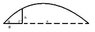# Finding the Spring Constant of a Water-Balloon Launcher

• Minte
In summary, the conversation is about finding the average spring constant of a water-balloon launcher and using it to determine the distance to pull back the launcher and launch a balloon of a certain weight a certain distance. The unknown variables are the spring constant (k) and the formula for it. The conversation also mentions using the formula for spring energy and kinetic energy to find the initial velocity and then using a standard 2D projectile motion calculation to find the horizontal flight distance. Eventually, the conversation is solved by using the formula k=Fwx/2BH and the teacher's formula.

## Homework Statement

Find the average spring constant (k) of a water-balloon launcher, and use that to find the distance to pull back the launcher and launch a balloon of a certain weight a certain distance. I'm at the "finding k" part.

Diagram attached.

Known:
Weight of balloon (Fw, N), mass of balloon (m, kg)
Height of launcher (H, m)
Length of pullback (B, m)
Angle Theta (since tan Th = h/B)
Flight distance / range (x, m)

Unknown:
Spring constant of launcher (k, N/m)

F=kx
U=kx^2/2
?

## The Attempt at a Solution

I just can't find a place to start substituting equations. I tried converting spring energy to gravitational / kinetic energy, but the formulas got very complicated very quickly and lots of extra variables (such as velocity, time, and acceleration) were introduced. I don't think that I can use Fw for Fs, otherwise, why would the pullback distance matter?

I have a lot of data (a whole day on the baseball field hurling water balloons worth), but I don't know how to find the spring constant! Once I have a formula, the rest will be a breeze, but there are too many options, which always makes my brain explode.

Solved
It turns out that the teacher had a formula that he wasn't giving us. k=Fwx/2BH, I think. Anyway, it has been solved. Thanks for the help, anyway! =D

#### Attachments

•phys.jpg
3.4 KB · Views: 373
Last edited:
It seems to me you could begin with spring energy = kinetic energy given to the balloon. That gives you the initial velocity as an expression with a k in it. From the initial v, you can do a standard 2D projectile motion calc to find the horizontal flight distance x in terms of k and the angle. This expression could be solved for k so you could calculate a value for v and then k from each of your test shots (where you know the angle and x).